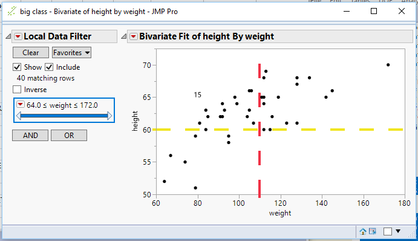Our World Statistics Day conversations have been a great reminder of how much statistics can inform our lives. Do you have an example of how statistics has made a difference in your life? Share your story with the Community!
Choose Language Hide Translation Bar
Highlighted

## How do I retrieve data from a bivariate fit

Is it possible to retrive data once you create the bivariate fit? I have reference lines and want to find the number of selected rows in each section.

At the moment, I am using selected rows with conditions such as x than or equal to 0 and y greater than 10 to find the number and then manually entering it onto the graph

I would like to select the data in each section and write the number to a text box within that section.

5 REPLIES 5
Highlighted

## Re: How do I retrieve data from a bivariate fit

The answer will depend on exactly how you have generated the reference lines, but it can definitely be done programmatically. Are you able to post a screen shot of your bivariate fit, or, better yet, some dummy data that is representative of what you have?

Highlighted

## Re: How do I retrieve data from a bivariate fit

This would be the code for the bivariate:

``````Bivariate(

Y( :Comb ),

X( :Quantity ),

Automatic Recalc( 1 ),

Local Data Filter(

Add Filter( columns( :Quanity), Where( :Quantity >= 0 & :Slack <= 897 ) )

),

SendToReport(

Dispatch( {}, "1", ScaleBox, {Add Ref Line( 0, "Dashed", "Red", "", 4 )} ),

Dispatch(

{},

"2",

ScaleBox,

{Add Ref Line( 25, "Dashed", "Medium Light Yellow", "", 4 )}

),

Dispatch( {}, "Bivar Plot", FrameBox, {Frame Size( 902, 512 )} )

)

)``````

What I am trying to do is when you use the local data filter it gives a value of matching rows in JMP. I then want to print that value into a text box on the graph without having to do it manually

I will have various local data filters eventually.

Highlighted

## Re: How do I retrieve data from a bivariate fit

Here is a modification to your code, using the sample data table, "Big Class", that calculates and places a count of the data points in one of the quadrants.``````Names Default To Here( 1 );
dt = Open( "\$SAMPLE_DATA/big class.jmp" );

Bivariate(
Y( :height ),
X( :weight ),
Automatic Recalc( 1 ),
Local Data Filter( Add Filter( columns( :weight ), Where( :weight >= 0 & :weight <= 200 ) ) ),
SendToReport(
Dispatch( {}, "1", ScaleBox, {Add Ref Line( 110, "Dashed", "Red", "", 4 )} ),
Dispatch( {}, "2", ScaleBox, {Add Ref Line( 60, "Dashed", "Medium Light Yellow", "", 4 )} ),
Dispatch( {}, "Bivar Plot", FrameBox, {Frame Size( 902, 512 )} ),
Dispatch(
{},
"Bivar Plot",
FrameBox,
{Frame Size( 411, 241 ), Add Graphics Script( 2, Description( "Script" ),
Text( Center Justified, {80, 65},
char(n rows(dt<<get rows where(:Height>60 & :Weight < 110) ) ))),
Grid Line Order( 1 ), Reference Line Order( 3 )})

)
);``````
Jim
Highlighted

## Re: How do I retrieve data from a bivariate fit

Would it be the exact same with Graph Builder?

Highlighted

## Re: How do I retrieve data from a bivariate fit

I would assume that it would be pretty close to this....however, there are many options in Graph Builder that could make it different.  All that I did to generate the JSL that I displayed, was to run the Bivariate, and the right click on the chart and choose "Customize".  I then built the Add Graphics Script from there.  So if you did the same with Graph Builder, you could get an idea of what syntax you will need for the modifications.

Jim
Article Labels

There are no labels assigned to this post.